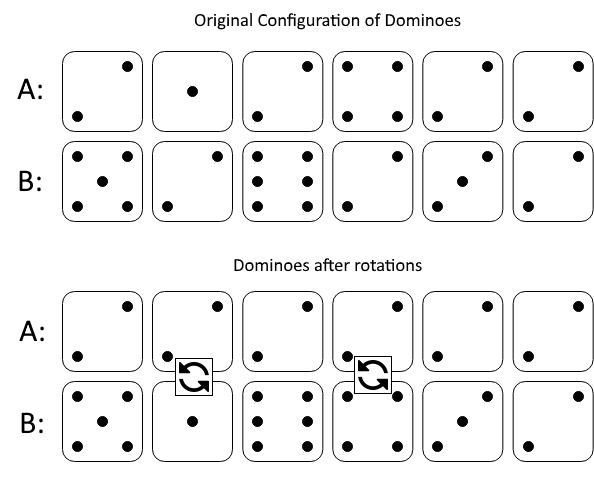##### Welcome to Subscribe On Youtube

Formatted question description: https://leetcode.ca/all/1007.html

# 1007. Minimum Domino Rotations For Equal Row

Medium

## Description

In a row of dominoes, A[i] and B[i] represent the top and bottom halves of the i-th domino. (A domino is a tile with two numbers from 1 to 6 - one on each half of the tile.)

We may rotate the i-th domino, so that A[i] and B[i] swap values.

Return the minimum number of rotations so that all the values in A are the same, or all the values in B are the same.

If it cannot be done, return -1.

Example 1:Input: A = [2,1,2,4,2,2], B = [5,2,6,2,3,2]

Output: 2

Explanation:

The first figure represents the dominoes as given by A and B: before we do any rotations.

If we rotate the second and fourth dominoes, we can make every value in the top row equal to 2, as indicated by the second figure.

Example 2:

Input: A = [3,5,1,2,3], B = [3,6,3,3,4]

Output: -1

Explanation:

In this case, it is not possible to rotate the dominoes to make one row of values equal.

Note:

1. 1 <= A[i], B[i] <= 6
2. 2 <= A.length == B.length <= 20000

## Solution

For each possible number from 1 to 6, store the indices of the number in A and B.

A number can be in all dominoes after rotations only if a number exists at every index in either A or B or both arrays. Count the numbers of occurrences of the number in A and B, and calculate the minimum number of rotations required, and return.

• class Solution {
public int minDominoRotations(int[] A, int[] B) {
Map<Integer, List<Integer>> map = new HashMap<Integer, List<Integer>>();
int length = A.length;
for (int i = 0; i < length; i++) {
int numA = A[i];
List<Integer> indicesA = map.getOrDefault(numA, new ArrayList<Integer>());
map.put(numA, indicesA);
int numB = B[i];
List<Integer> indicesB = map.getOrDefault(numB, new ArrayList<Integer>());
map.put(numB, indicesB);
}
int minRotation = Integer.MAX_VALUE;
Set<Integer> keySet = map.keySet();
for (int key : keySet) {
List<Integer> indices = map.getOrDefault(key, new ArrayList<Integer>());
Set<Integer> indicesSet = new HashSet<Integer>();
for (int index : indices)
if (indicesSet.size() == length) {
Collections.sort(indices, new Comparator<Integer>() {
public int compare(Integer num1, Integer num2) {
return Math.abs(num1) - Math.abs(num2);
}
});
int countA = 0, countB = 0;
int size = indices.size();
for (int i = 0; i < size; i++) {
int index = indices.get(i);
if (index > 0)
countA++;
else
countB++;
}
int curMinRotation = length - Math.max(countA, countB);
minRotation = Math.min(minRotation, curMinRotation);
}
}
return minRotation == Integer.MAX_VALUE ? -1 : minRotation;
}
}

• class Solution:
def minDominoRotations(self, A: List[int], B: List[int]) -> int:
a, b = A, B
c, d = b, a
counta, countb = 0, 0
countc, countd = 1, 1
for ai, bi in zip(A[1:], B[1:]):
if ai == a:
pass
elif ai != a and bi == a:
counta += 1
else:
counta = -30000
if bi == b:
pass
elif bi != b and ai == b:
countb += 1
else:
countb = -30000
if ai == c:
pass
elif ai != c and bi == c:
countc += 1
else:
countc = -30000
if bi == d:
pass
elif bi != d and ai == d:
countd += 1
else:
countd = -30000
if counta < 0 and countb < 0 and countc < 0 and countd < 0:
return -1
else:
ans = 30000
for count in [counta, countb, countc, countd]:
if count >= 0:
ans = min(ans, count)
return ans

############

class Solution(object):
def minDominoRotations(self, A, B):
"""
:type A: List[int]
:type B: List[int]
:rtype: int
"""
N = len(A)
count = collections.Counter(A + B)
if count.most_common(1) < N:
return -1
target = count.most_common(1)
a_swap = 0
b_swap = 0
for i in range(N):
if A[i] == B[i]:
if A[i] == target:
continue
else:
return -1
elif A[i] == target:
b_swap += 1
elif B[i] == target:
a_swap += 1
else:
return -1
return min(a_swap, b_swap)